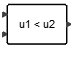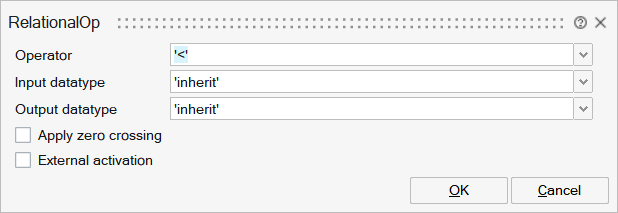# RelationalOp

This block compares two input signals defined by the relational operator (parameter).## Library

LogicalOperations

## Description

The RelationalOp block compares two input signals defined by the relational operator specified by block parameter. The comparision is made elementwise. The elements of the output matrix signal are either TRUE or False, represented by 1 and 0, respectively.

Supported relational operations are : "==", "~=", "<", "<=", ">" and ">=".

if the operator is "==", the result is TRUE if the two inputs are equal.

if the operator is "~=", the result is TRUE if the two inputs are not equal.

if the operator is "<", the result is TRUE if the first input is less than the second input.

if the operator is "<=", the result is TRUE if the first input is less or equal than the second input.

if the operator is ">", the result is TRUE if the first input is greater than the second input.

if the operator is ">=", the result is TRUE if the first input is greater or equal than the second input.

## ParametersNameLabelDescriptionData TypeValid Values

rule

Operator

Relational operations supported by the RelationalOp block are : "==", "~=", "<", "<=", ">" and ">=".

String

'=='
'~='
'<'
'<='
'>'
'>='

typei

Input datatype

Defines the datatype of the inputs.
Supported types are : double, int32, int16, int8, uint32, uint16, uint8, boolean, inherit.

String

'double'
'complex'
'int32'
'int16'
'int8'
'uint32'
'uint16'
'uint8'
'boolean'
'inherit'

typeo

Output datatype

Defines the datatype of the output.
Supported types are : double, int32, int16, int8, uint32, uint16, uint8, boolean, inherit.

String

'double'
'complex'
'int32'
'int16'
'int8'
'uint32'
'uint16'
'uint8'
'boolean'
'inherit'

zcr

Apply zero crossing

Parameter to activate zero-crossing detection.

Scalar

0
1

externalActivation

External activation

This parameter defines if the block receives an external activation or inherits. When External Activation is used, an activation port is added to the block.

Number

0
1

## Ports

NameTypeDescriptionIO TypeNumber

Port 1

explicit

input

1

Port 2

explicit

input

2

Port 3

explicit

output

1

Port 4

activation

input

externalActivation

NameValueDescription

always active

no

direct-feedthrough

yes

zero crossing

no

mode

no

continuous-time state

no

discrete-time state

no